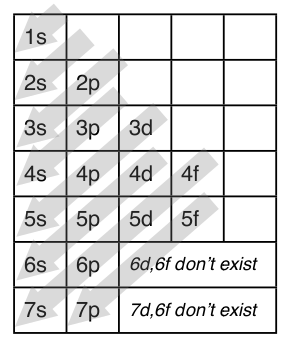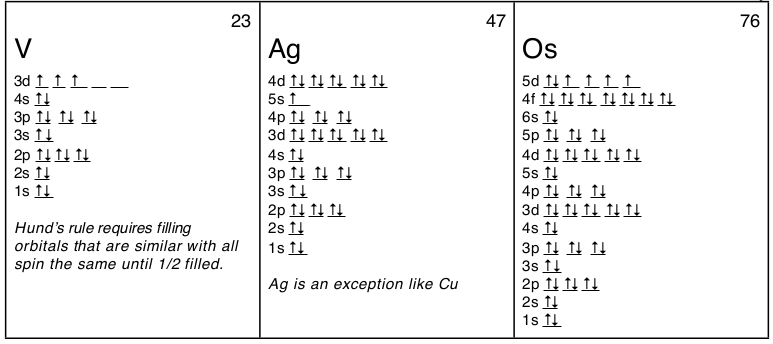# 1.4: Electron Configuration and Orbital Diagrams

•• Contributed by Kenneth Pringle
• EssentialChemistryTextbook at Curriki

## Electron Configuration

The purpose of introducing quantum numbers has been to show that similarities in the electron arrangement or electron configuration lead to the similarities and differences in the properties of elements. But writing the quantum numbers of electrons of an element in set notation like {2,1,-1,1⁄2} is time consuming and difficult to compare so an abbreviated form was developed. An electron configuration lists only the first two quantum numbers, n and $$\ell$$, and then shows how many electrons exist in each orbital. For example, write the electron configuration of scandium, Sc: 1s2 2s2 2p6 3s2 3p6 4s2 3d1 . So for scandium the 1st and 2nd electron must be in 1s orbital, the 3rd and 4th in the 2s, the 5th through 10th in the 2p orbitals, etc.This is a memory device to remember the order of orbitals for the first two quantum numbers. Follow the arrow starting in the upper right, when the arrow ends go to the next arrow and start again.

In Scandium, the 4s has lower energy and appears before 3d (the complexity of the d-orbitals leads to its higher energy), so it is written before adding 3d to the electron configuration. But it is common to to keep all the principle quantum numbers together so you may see the electron configuration written as Sc: 1s2 2s2 2p6 3s2 3p6 4s2 3d1. Writing electron configurations like this can cause difficulties in determining the element that matches an electron configuration. But if you just count the number of electrons it will equal the number of protons which equals the atomic number which is unique for each element. For example: “Which element has the electron configuration: 1s2 2s2 2p6 3s2 3p6 4s2 3d104s24p6 4d8 5s2 ?” Counting the electrons gives 46, which is the atomic number of palladium.

Here’s a diagram of the first several electron configurations. David’s Whizzy Periodic Table is a visual way of looking at the changing electron configuration of elements.Note the 3d orbital follows the 4s in the lowest row, but starting with Ga (#31) it is next to the 3p orbital. It is most commonly listed with the other 3 orbitals, but sometimes it follows the 4s orbital to indicate that the 3d orbital is lower in energy than the 4s while it is being filled.

## Periodic Table Exceptions To Know

There is a major exception to the normal order of electron configuration at Cr (#24) and Cu (#29). It turns out that the energy the electron configuration that is half-filled, 4s1 3d5 , and filled orbital, 4s1 3d10 , has lower energy than the typical filling order, 4s2 3d4 , and 4s2 3d9 . This pattern is followed in the 5th row with Mo (#42) and Ag (#47).

## f block

Elements For completeness a couple of f-block elements are listed here. Neodymium, Nd, which is used in very powerful magnets, has an atomic number of 60. For 60 electrons the electron configuration is:

1s2 2s2 2p6 3s2 3p6 4s2 3d104s24p6 4d105s25p66s24f4

For californium, Cf, with 98 electrons the electron configuration is:

1s2 2s2 2p6 3s2 3p6 4s2 3d104s24p6 4d105s25p66s24f145d106p67s25f10

## Orbital Diagrams

Many times it is necessary to see all the quantum numbers in an electron configuration, this the purpose of the orbital diagram. In addition to listing the principle quantum number, n, and the subshell, $$\ell$$, the orbital diagram shows all the different orientations and the spin of every electron. The diagram shows the number of subshell by using boxes or lines for electrons (use three for p-orbitals, five for d-orbitals, and 7 for f-orbitals). In each box the spin of an electron is noted by using arrows, up arrows mean 1⁄2 spin and down arrows mean –1⁄2 spin. For example, the orbital diagram for the first 18 atoms are shown below.## Rules for Filling Orbitals

• Aufbau Principle states that the lowest energy orbital is filled first. So electrons usually fill the lowest energy level and the simplest orbital shape first.
• Pauli Exclusion Principle states that no two electrons can have the same four quantum numbers. This is why each orbital only has two electrons, one spin up ( 1⁄2) and one spin down (–1⁄2).
• Hund’s Rule states that orbitals of the same energy, those which differ only in their orientation, are filled with electrons with the same spin before the second electron is added to any of the orbitals. This is why electrons have up spin, ↑, in the orbital diagrams of B to N and of Al to P in the diagrams above.

## More Examples of Orbital Diagrams

Here are some orbital diagrams of elements with more electrons to help you understand the rules, electron configuration, orbital diagrams, and quantum numbers.## Noble Gas Abbreviation

Writing out the electron configuration over and over can be tedious and shifts ones attention away from the outer electrons which are the most important electrons. So an abbreviated form of electron configurations was developed using the final column of the periodic table, the noble gases. Any element can be abbreviated except H and He, by using the noble gas with fewer electrons than the element. For example, instead of Sc : 1s22s22p63s23p64s23d1 it would be abbreviated as [Ar]4s23d1 or [Ar]3d14s2. For Ag the abbreviation would be: [Kr]5s14d10 (see orbital diagram above), and for Os: [Xe]6s24f145d6 or [Xe]4f145d66s2. Just remember that the abbreviations require that you use oble gases only and that you use a noble gas with fewer electrons. Also you can’t abbreviate a noble gas by using its symbol in brackets; that is, Ar is [Ne]3s23p6 not [Ar]. Finally, you can still count the number of electrons to determine the element, you just start with the number of electrons in the noble gas. For example “What is the element with the electron configuration: [Xe]6s24f145d6 ?” Counting the electrons 54 + 2 + 14 + 6 = 76 which is the atomic number for osmium, Os.

## Similarities in Electron Configuration

Equal Similar Properties. Now we can put together the first and second part of this unit. When the periodic table was being developed, chemists looked for similarities in chemical and physical properties. Any theory that describes the arrangement of electrons must be able to explain these similarities. Let’s look at the electron configurations in a periodic table format again.The first column of this periodic table has a single electron in the outer s-orbital: H 1s1, Li 2s1, Na 3s1 , K 4s1. So that similarity in the electrons in the outer energy level must be why the alkali metals are all acting the same both in their chemistry and their physical properties. Hydrogen is an exception because it is a single proton in the nucleus and single electron which gives it wholly unique properties (although at high pressure and low temperature it can act as a metal). All the way through the periodic table we see this same pattern in each column where the outermost electrons have filled the subshells in a similar manner. For transition metals the outermost electrons are the 4s2 electrons that surround the filling 3d orbital (the 4s is in the 4th energy level and the 3d is in the lower 3rd energy level). As transition metals add more electrons and more protons their properties change more subtly than the alkali metals and alkaline earth metals, because the outermost electrons are nearly always the same (remember the exceptions of Cr and Cu). The outermost electrons are so important that we give them a name: valence electrons. The valence electrons will be a major part of our discussion of bonding and formation of compounds.